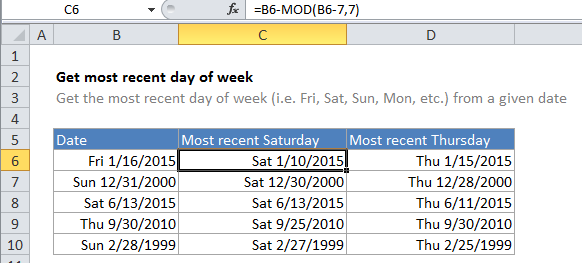## Excel Office

Excel How Tos, Tutorials, Tips & Tricks, Shortcuts

# How to calculate most recent day of week in Excel

If you want to find the most recent Wednesday, or Saturday, or Monday, given a specific start date, you can use a formula that uses the MOD function.

## Formula

`=date-MOD(date-dow,7)`Note: If the the date has the same day of week, date will be returned.

## Explanation

Formulas that use the concept of “day of week” are based on the idea that each day of of the week has a specific number. By default, Excel assigns 1 to Sunday and 7 to Saturday.

Also See:   MOD function: Description, Usage, Syntax, Examples and Explanation

In the example formula, B6 is the date 1/16/2015, and the formula in C6 is:

`=B6-MOD(B6-7,7)`

The number 7 (the number argument in the MOD function) represents the day of week (dow) that you want.

To solve this formula, Excel first subtracts the dow (7 in this case) from the date, then feeds the result into the MOD function as the number. MOD returns the remainder of dividing that number by 7, which is then subtracted from date.

```=B6-MOD(B6-7,7)
=B6-MOD(42013,7)
=B6-6
=42014
=1/10/2015```

If you want to get the most recent day of week from the current date, you can use the TODAY function, like so:

`=TODAY()-MOD(TODAY()-dow,7)`

Also See:   Max of every nth column in Excel